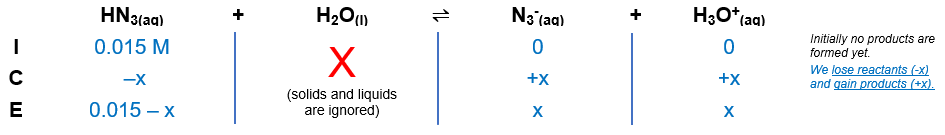# Problem: What is the pH of a 0.015 M aqueous solution of hydrazoic acid (HN3) (Ka = 1.9 x 10–5) at 25°C?a. 2.89b. 1.82c. 3.28d. 6.55e. 4.87

###### FREE Expert Solution

Equilibrium reaction:        HN3(aq) + H2O(l)  N3-(aq) + H3O+(aq)

Step 1: Construct an ICE chart for the reaction.Step 2: Write the Ka expression.

$\overline{){{\mathbf{K}}}_{{\mathbf{a}}}{\mathbf{=}}\frac{\mathbf{products}}{\mathbf{reactants}}}\phantom{\rule{0ex}{0ex}}{\mathbf{K}}_{\mathbf{a}}\mathbf{=}\frac{\mathbf{\left[}{{\mathbf{N}}_{\mathbf{3}}}^{\mathbf{-}}\mathbf{\right]}\mathbf{\left[}{\mathbf{H}}_{\mathbf{3}}{\mathbf{O}}^{\mathbf{+}}\mathbf{\right]}}{\left[{\mathrm{HN}}_{3}\right]}$

Step 3: Calculate the equilibrium concentrations.

${\mathbf{K}}_{\mathbf{a}}\mathbf{=}\frac{\left[\mathbf{x}\right]\left[\mathbf{x}\right]}{\left[\mathbf{0}\mathbf{.}\mathbf{015}\mathbf{-}\mathbf{x}\right]}\phantom{\rule{0ex}{0ex}}\mathbf{1}\mathbf{.}\mathbf{9}\mathbf{×}{\mathbf{10}}^{\mathbf{-}\mathbf{5}}\mathbf{=}\frac{{\mathbf{x}}^{\mathbf{2}}}{\mathbf{0}\mathbf{.}\mathbf{015}\mathbf{-}\mathbf{x}}$

94% (57 ratings)###### Problem Details

What is the pH of a 0.015 M aqueous solution of hydrazoic acid (HN3) (Ka = 1.9 x 10–5) at 25°C?

a. 2.89
b. 1.82
c. 3.28
d. 6.55
e. 4.87